# String Length JavaScript (THE Ultimate Masterpost)

In this post, we’ll be looking at different ways to check string length in Javascript. You can either use the pre-defined string functions/properties in Javascript or create a manual function of your own. Let’s look at how to do both in this article.

## Javascript’s length property

Javascript offers a basic string length property to calculate the length of the string. If you don’t want to understand the inner-workings of how finding string lengths work, just stick to this property, and you’re done.
The syntax for this property is as follows:

`string.length`

In the above syntax, “string” is the name of the string you’ve declared. By affixing the name of the string with the property “length”, after a period, you get your string length.

You’ll get an integer value as the result (whole number). For example, if a string has 10 characters, the value of string.length will now be 10.

You can assign this value to a new variable so you can use it down the line.

Let’s look at an example to see how this property works in real time.

Let me create a variable “a” and assign it a string “Hello World”.

`var a = "Hello World";`

Then, let me create another variable “len” to hold the integer value generated when I use the length property to find the length of “a”.

Then, let me ask the browser to calculate the length of “a” using the pre-defined Javascript property “length” and assign that value to “len”.

`var len = a.length;`

Now that “len” holds the actual length of the string “a”, let me display that on the screen.

`document.write("Length of the string is " + len);`

This will be the output of the above program: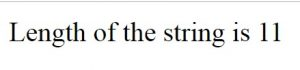The length of the string is displayed as 11, even though the number of characters in the word “Hello” is 5 and “World” is 5, so together, the number of characters in the word “Hello World” is 10.

But Javascript calculates spaces as characters as well, and since I’ve created a space between “Hello” and “World”, the string length here is 11, and not 10.

If I create 2 spaces between “Hello” and “World”, then the string length will change to 12.

So, spaces and special characters are also considered a part of the string length.

### Calculating string length for strings with extra spaces

Ok, now we know that spaces in between words will be taken in account while calculating string length, but what about spaces before or after a string?

To the human eye, it seems redundant to calculate extra spaces of any kind into a string length, but does Javascript see things like we do? Let’s test and find out.

`var a = " Hello World ";`

Now, I’ve created 2 extra spaces, one at the start and another one at the end of the string.

When I calculate and display the string length value for this new string, this is what I get: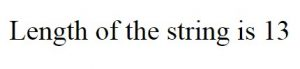Do you see how the string length value has increased by two? This is in accordance with the 2 extra spaces I just added.

So, Javascript does not considered extra spaces as just that, extras that shouldn’t be taken into consideration.
There are ways to get around this, like using the build-in trim() function to trim out extra spaces, but that’s for another post.

## Using the string length value to make comparisons

So now you know how string length works and how to use the length property to find the same. But how exactly would you use this value? What’s its use?

Well, for one, you could use the string length property to compare two strings. You could check if the lengths of two strings are the same, find the longer string, and so on.

The best way to do string comparisons, especially length comparisons is by using the if loop.

Let’s look at an example of how to do the same.

Let’s create two variables and assign two strings to them.

```var a = "Hello World";
var b = "Bonjour everyone";```

Now, let’s find the length of these strings and compare them. I’m going to be using the length properties directly because you don’t necessarily have to assign them to a separate variable to be able to use them.

```if(a.length > b.length) {
document.write("String a is longer than string b");
}
else {
document.write("String b is longer than string b");
}```

If I run this program, this is what I get.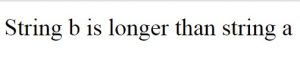So, string “a” has a length of 11, including the space between the words. String “b” on the other hand has a string length of 16.

So, when the if condition runs, it’ll first test the condition:

`a.length < b.length`

This will return a false, since 11 < 16 is false. So, the browser will execute the else condition and display the result you see in the above screenshot. So, this is one of the best ways of using string lengths in programs.

## Using the string length value in loops

You can also use string lengths in loops. Let me show you how.

Let’s look at a for loop first. It’s usually written as follows:

```for(var i=0; i<10; i++) {
document.write(i + "<br\>");
}```

You’ll get the following output for the above statement: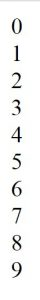So, what happens here is, the for loop is run 10 times, from 0 to 9 and it stops just as the “i” value becomes 10, because the condition statement says that i should be less than 10, not less than and equal to 10.

So, the number 10 can be replaced with a variable too, like this:

```var a = 10;
for(var i=0; i<a; i++) {
document.write(i + "<br\>");
}```

We’ll get the same result as seen in the previous screenshot when we run the above program. The only difference between the 2 programs is that the value 10 is assigned to a variable “a” and we’ve used that in the for loop instead of the number.

Similarly, we can create a string, calculate its length and use that value in the condition statement because string length calculations produce integer numbers.

Let’s see how that works.

```var a = "Hello World";
for(var i=0; i<a.length; i++) {
document.write(i + "<br\>");
}```

In the above program, I’ve created a variable “a” and assigned it a string “Hello World”.

Then, I’ve calculated its length using Javascript’s length property and used that in the condition statement of the for loop.

This is the result I get when I run the above program: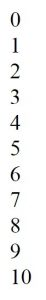So, what happens here is, the value of a.length is first calculated by the browser. We know it’s 11, since the browser will calculate the space as well.

So, the statement i < a.length would now become i < 11. The for loop will now run from i = 0 through i = 10, and stop execution when i becomes 11. This is how you can use string length calculations in loops. The same goes for a while loop as well. Let me show you: [js]var a = "Hello World"; var i=0; while (i < a.length) { document.write(i + "<br/>"); i++; }[/js] The above program will display the same result as the program that used the for loop as well.

## Finding the string length using array methods

To execute this method properly, you need to first split the string into an array, so each character in the string becomes an array item.

Then, you can calculate the length of the array, which will be equal to the length of the string.

Let’s see how this works:

```var a = "Hello World";
var ar = a.split('');
document.write(ar);```

In the above program, I’ve first declared the variable “a” and assigned it a string “Hello World”.

Then, I’ve used Javascript’s built-in split() method to split the string into an array, and assigned it to the variable “ar”.

The split method takes an argument, which is an empty string in this case, and it’ll split the give string based on that.

So, in this case, since we’ve given the argument as an empty string, the function will go through the entire string and split each character into a separate array item.

This will be the output.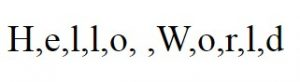Do you see how the space between the two words is also assigned a place in the array?

Now, let’s find the length of this array and display it.

`document.write("Length of this string is " + ar.length);`

Arrays use the length property as well, so once we’ve converted out string into an array, we can use the length property to find the length of that array.

This will be the output: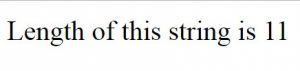As I said, this is a roundabout way of going about finding the length. You are better off using the string length property, but I just wanted to teach you how to do the same with arrays.

## Finding the string length using manual methods (no native Javascript function)

Ok, so far, we’ve been looking at Javascript’s pre-defined functions for finding the length of strings. I agree that using the length property is the easiest and most efficient way of finding the string length.

But I also believe that it’s always good to know the manual way of solving a given problem. If you resort to shortcuts in every case, you’ll never learn the process.

So, let’s look at the manual way of achieving the same. I’m going to try to find the string length without using any Native Javascript functions.

In order to do that, I’ll first be creating a function definition so we can call that function whenever we want the length of the string found.

So, this is how the algorithm works:
2. Create a variable “len” to store the length and assign it an initial value of 0.
3. Then, create a loop that loops over the string until the loop runs out of the characters in the string.

That’s it. Let’s execute this algorithm in code now.

```function stringLen(str) {
var len = 0;
while(str[len] != undefined) {
len++;
}
return len;
}```

Look at the above code. It receives the string as “str”.

Then, it creates a variable “len” and assigns it an initial value 0.

The, using the while loop, we’ll loop through the string with the condition str[len] != undefined.

Strings are basically arrays strung together.

Let’s say the string is “Hello”.

Then, str will be “H”.

Str will be “e” and so on.

So, when we reach str, which is “o”, we’d have reached the end of the string.

But the len value will still increment and become 5.

When the while loop checks for str though, it’ll get an “undefined” because the string has run out of characters.

Now, it’ll return 5 back to where the function call came from.

This is why we started the len value from 0, and not 1. Otherwise, we’d end up with a higher value than the actual length of the string.

Let’s call the above function and check if it works now:

`document.write(stringLen("Hello World"));`

The output for the above function call would be as follows: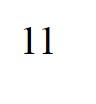As you can see, it works. It calculates the length of the string and gives the same result as the string length property.

We created the above program with the while loop. But is it possible to achieve the same result with the for loop? Let’s test and find out.

```function stringLen(str) {
for(var len = 0; str[len] != undefined; len++) {

}
return len;
}```

We don’t need to create any lines of code inside the for loop because our only objective here is the increment the len value.

Let’s test the output now.

`document.write("For loop: " +stringLen("Hello World"));`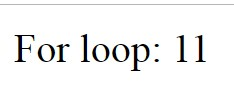We end up with the same length value as before, so it works with both while and for loops.

So that’s it. I tried to make this article an extensive source of various methods to find the length of a string in Javascript, and I hope I achieved that objective.